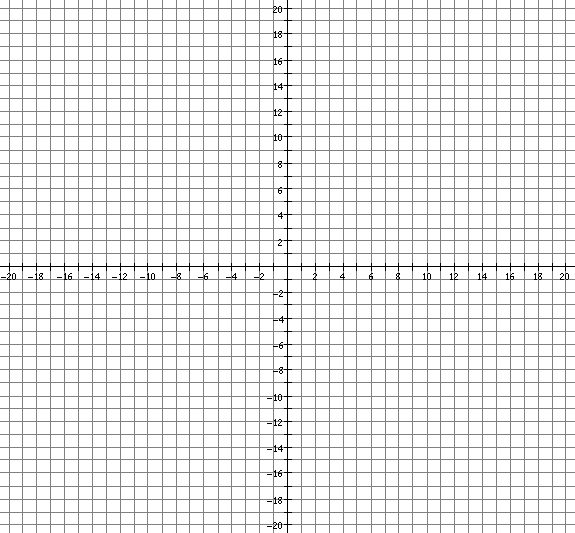Graphing Systems of Equations
Graphing Systems of Equations to Solve Them
Put the equations in one of the forms below and solve the system of equations graphically.

Three forms of equations are:
1. Slope-intercept form:     y = mx + b
(Solve each equation for y and then use the slope and y-intercept).
2. Standard form:     Ax + By = C
(For each equation plot the x-intercept and y-intercept and draw the line through them).
3. Point-slope form:     y - y1 = m(x - x1)
(Plot the given point and use the slope to find the next point).

Problem # 1